﻿ 联合外形响应的深度目标追踪器
«上一篇文章快速检索 高级检索

 智能系统学报2019, Vol. 14Issue (4): 725-732  DOI: 10.11992/tis.2018070290

### 引用本文SUN Haiyu, CHEN Xiuhong, XIAO Hanxiong. A deep object tracker with outline response map[J]. CAAI Transactions on Intelligent Systems, 2019, 14(4): 725-732. DOI: 10.11992/tis.201807029.### 文章历史

A deep object tracker with outline response map
SUN Haiyu, CHEN Xiuhong , XIAO Hanxiong
School of Digital Media, Jiangnan University, Wuxi 214122, China
Abstract: When convolutional neural network is used as a template to locate target, noise may be unavoidable in the final location response. To solve this problem, we developed a deep object tracker by combining the convolutional position response with the outline position response. For example, in the current frame, after extracting convolutional features and the outline information from the predicted target in the previous frame, we obtained the corresponding convolutional position response and the outline position response, and the latter was used to rectify the former in controlling the noise generated in the convolutional position response. The favorable results of our deep tracker on the benchmark show that the method of integrating the outline position response into the convolutional position response can greatly improve the precision and accuracy of the tracker.
Key words: object tracking    neural network    convolutional features    correlation filter    position response    outline information    noise suppression    rectify    deep learning

1 模板匹配以及外形信息

1.1 孪生网络追踪器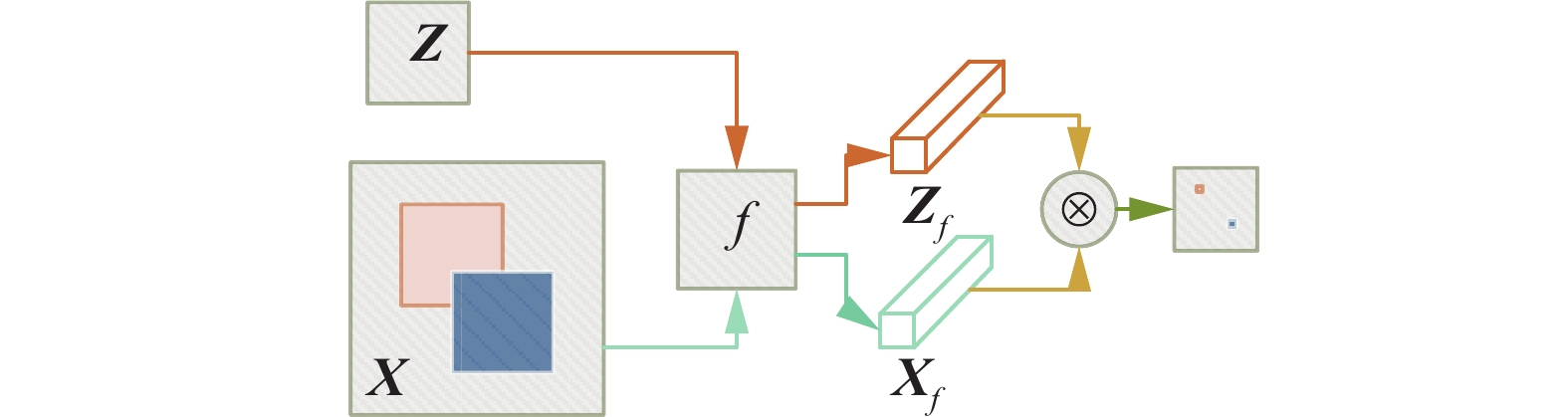Download: 图 1 孪生网络追踪器结构图 Fig. 1 Siamese network tracker structure
1.2 相关滤波追踪器

 $l = {\left\| {\sum\limits_{i = 1}^d {{{{H}}^i} \cdot {{{F}}^i} - {{G}}} } \right\|^2} + {\textit{λ}} \sum\limits_{i = 1}^d {{{\left\| {{{{H}}^i}} \right\|}^2}}$ (1)

 ${{{H}}^i} = \frac{{{{\cal{G}}_*} \circ {{\cal{F}}^i}}}{{\sum\limits_{k = 1}^d {{\cal{F}}_*^k \circ {{\cal{F}}^k} + \lambda } }}$ (2)

 ${{A}}_t^i = \left( {1 - \alpha } \right) {{A}}_{t - 1}^i + \alpha {\cal{G}}_*^t \circ {\cal{F}}_t^i$ (3)
 ${{B}}_t = \left( {1 - \alpha } \right){{B}}_{t - 1} + \alpha \sum\limits_{k = 1}^d { {\cal{F}}_{t * }^k { \circ {\cal{F}}}_t^k }$ (4)

 ${{P}} = D^{ - 1} \left\{ {\frac{{\sum\limits_{i = 1}^d {{{A}}_ * ^i \circ {{Z}}^i } }}{{{{B}} + {\textit{λ}}}}} \right\} = D^{ - 1} \left\{ {{{{H}}_ * } \circ {{Z}}} \right\}$ (5)

1.3 目标外形信息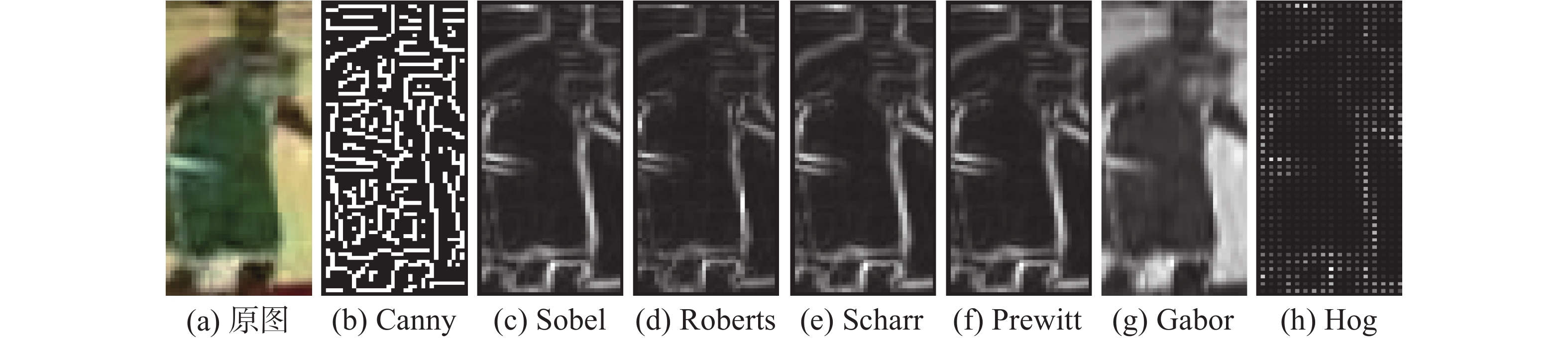Download: 图 2 外形信息检测方法 Fig. 2 The samples of methods to detect outlines
2 联合外形响应的深度目标追踪器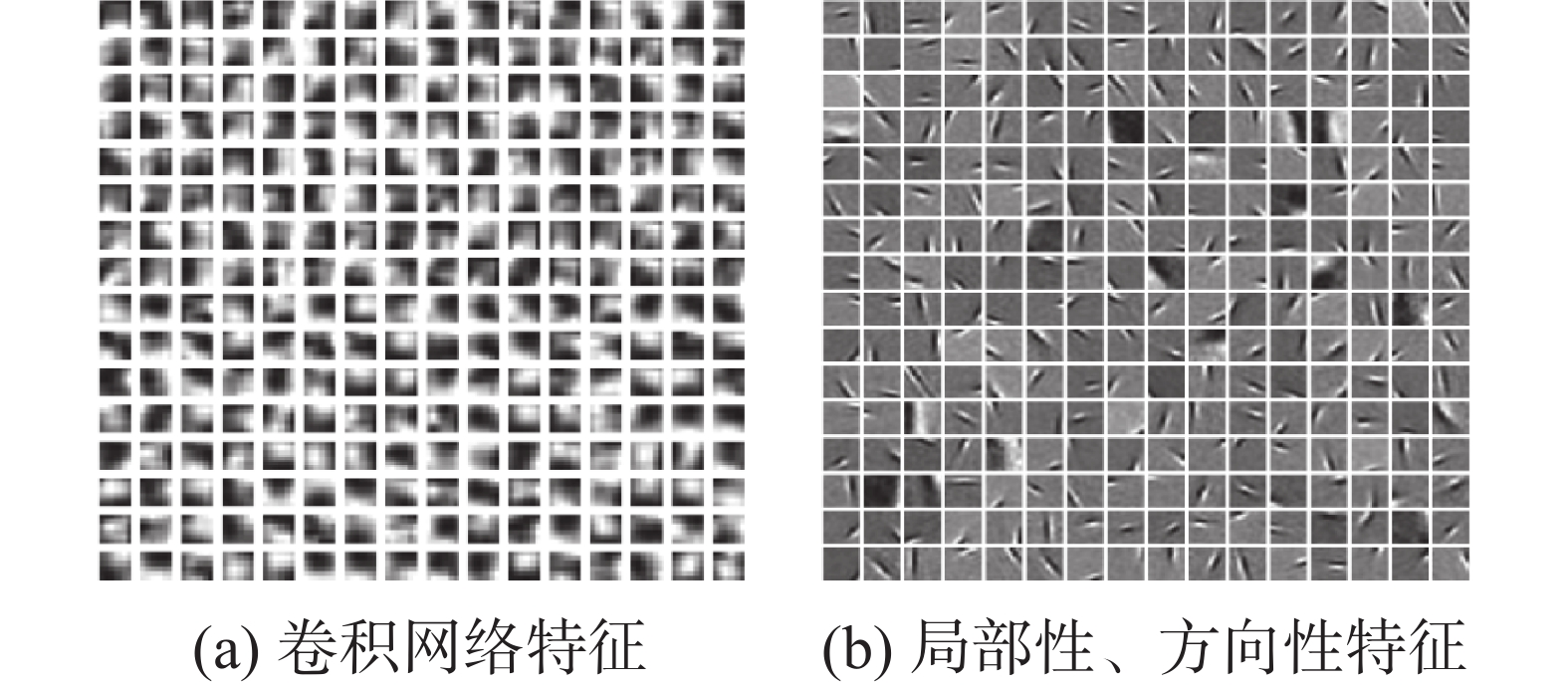Download: 图 3 特征对比 Fig. 3 Features comparison
2.1 网络结构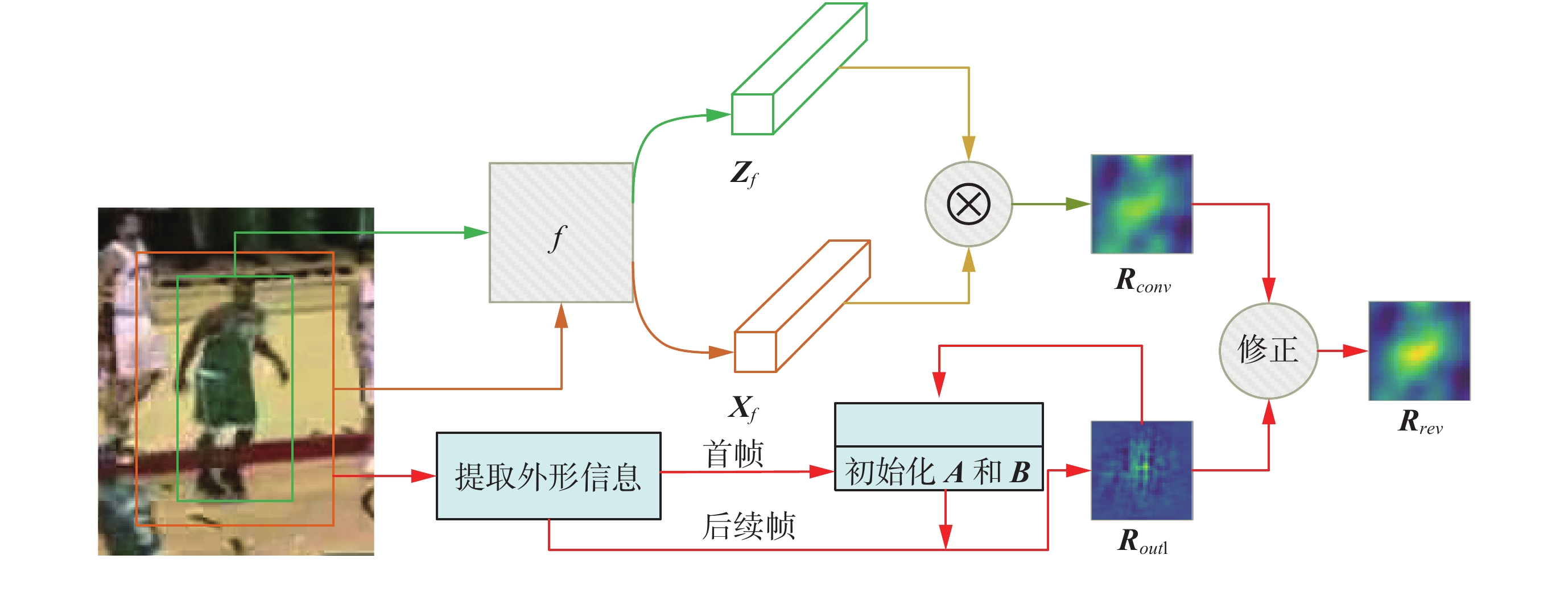Download: 图 4 本文目标追踪器结构 Fig. 4 The architecture of the proposed deep tracker
2.1.1 卷积匹配部分

2.1.2 滤波匹配部分

2.2 修正部分

 ${{R}}_{{\rm{rev}}} = \eta {{R}}_{{\rm{conv}}} + \left( {1 - \eta } \right) {{R}}_{{\rm{outl}}}$ (6)

 ${{{R}}_{{\rm{norm}}}} = \frac{{{{{R}}_{{\rm{old}}}}}}{{\max \left( {{{{R}}_{{\rm{old}}}}} \right)}}$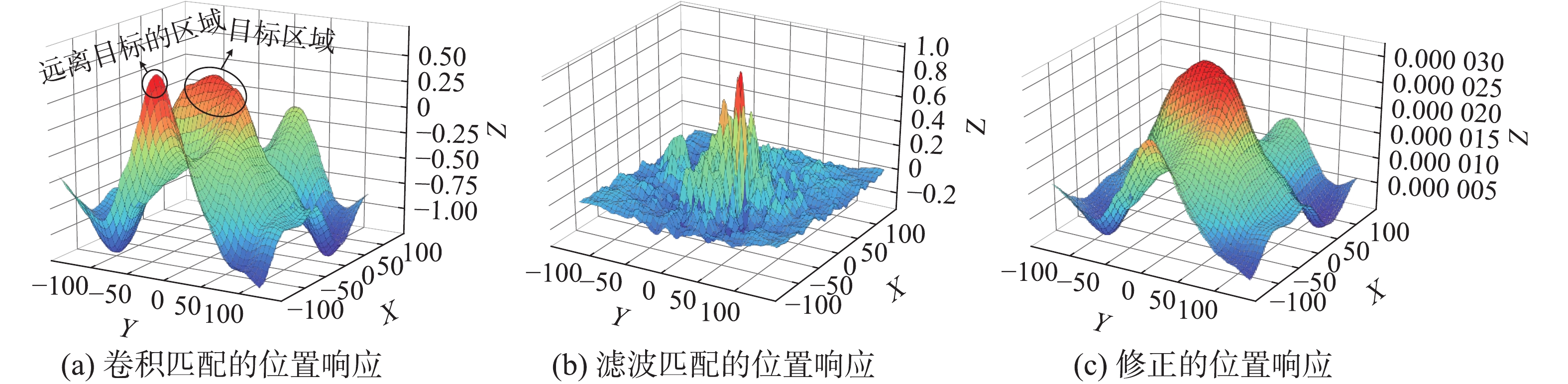Download: 图 5 位置响应的变化 Fig. 5 The transform of position response map
3 实验配置以及评价指标 3.1 实验细节

 $\begin{split} {l_{{\rm{train}}}} = & {N^{ - 1}}\sum\limits_{i, j} {{{{G}}_{ij}} \times \left[ { - \ln \left( {\operatorname{sigmoid} \left( {{{R}}_{{\rm{conv}}}^{ij}} \right)} \right)} \right]} + \\ & \left( {1 - {{{G}}_{ij}}} \right) \times \left[ { - \ln \left( {1 - {\rm sigmoid}\left( {{{R}}_{{\rm{conv}}}^{ij}} \right)} \right)} \right] \end{split}$

 ${\rm{sigmoid}}\left( x \right) = \frac{1}{{1 + \exp \left( { - x} \right)}}$

 $l = {l_{{\rm{init}}}} \times {r^{\displaystyle\frac{{{s_{{\rm{global}}}}}}{{{s_{{\rm{decay}}}}}}}}$

 $g = \exp \left( {\frac{{{x^2} + {y^2}}}{{2{\sigma ^2}}}} \right)$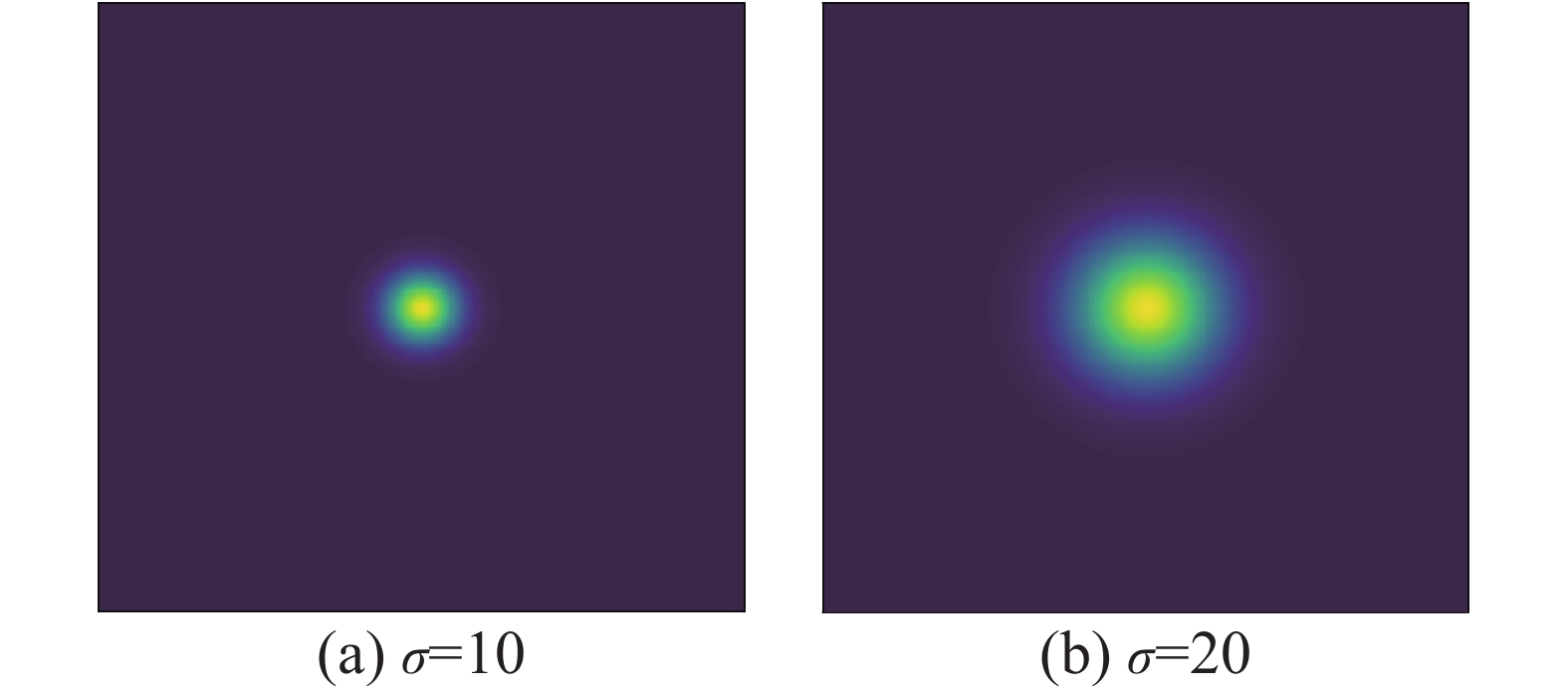Download: 图 6 $\sigma$ 效果图 Fig. 6 The effect of $\sigma$
3.2 实验设备

3.3 测试数据集以及评价指标 3.3.1 指标

 $o = \frac{{ {{A}}_{{\rm{track}}} \cap {{A}}_{{\rm{groundtruth}}} }}{{ {{A}}_{{\rm{track}}} \cup{{A}}_{{\rm{groundtruth}}} }}$

3.3.2 测试数据集

3.3.3 外形信息的时间花费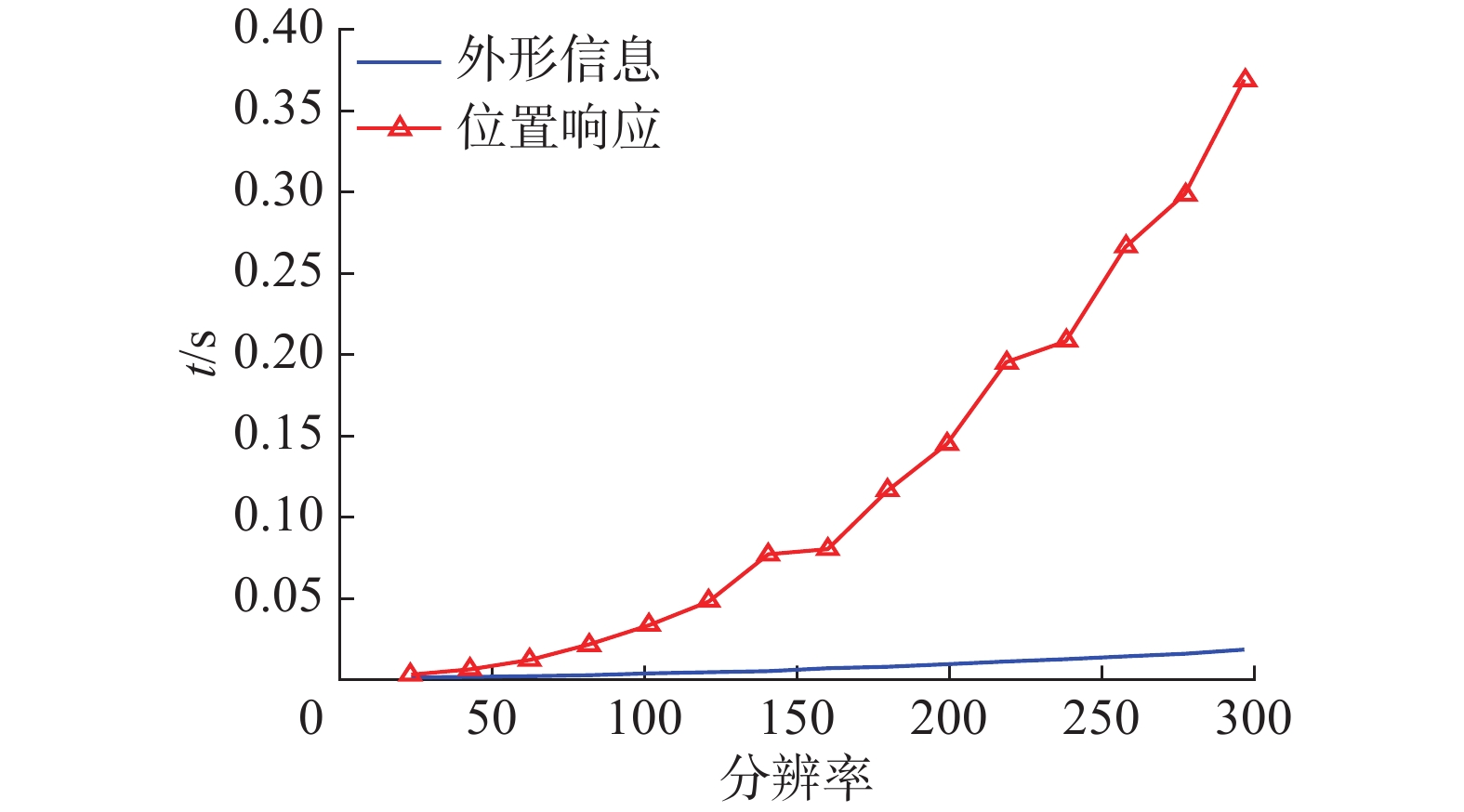Download: 图 7 时间花费 Fig. 7 The results of elapsed time
3.4 平台测试结果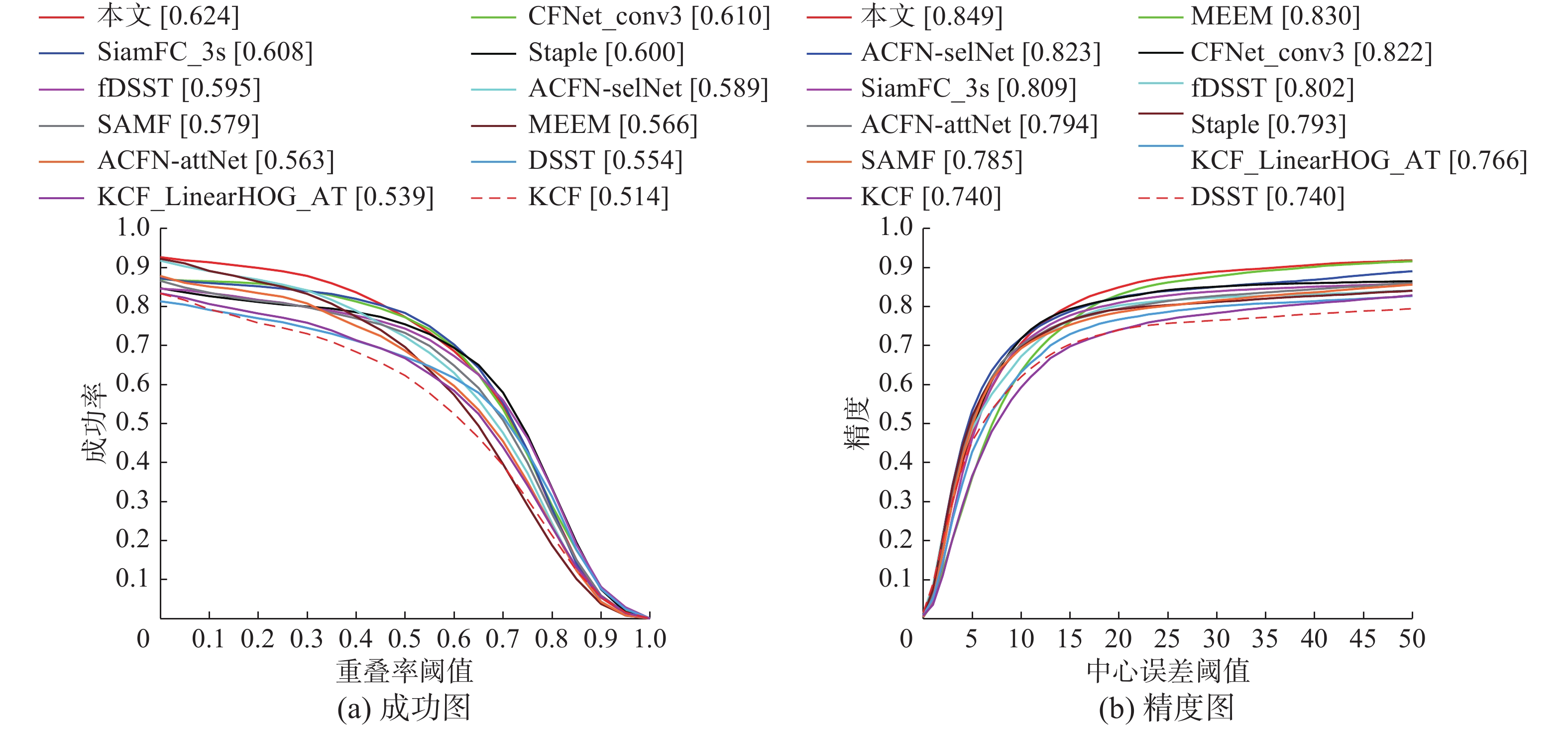Download: 图 8 测试结果 Fig. 8 The results of object tracking benchmarkDownload: 图 9 追踪效果直观感受 Fig. 9 The direct feeling of our tracker
3.5 直观效果

4 结束语

  WU Yi, LIM J, YANG M H. Online object tracking: a benchmark[C]//Proceedings of 2013 IEEE Conference on Computer Vision and Pattern Recognition. Portland, USA, 2013: 2411–2418. (0)  杨戈, 刘宏. 视觉跟踪算法综述[J]. 智能系统学报, 2010, 5(2): 95-105. YANG Ge, LIU Hong. Survey of visual tracking algorithms[J]. CAAI transactions on intelligent systems, 2010, 5(2): 95-105. DOI:10.3969/j.issn.1673-4785.2010.02.001 (0)  WU Yi, LIM J, YANG M H. Object tracking benchmark[J]. IEEE transactions on pattern analysis and machine intelligence, 2015, 37(9): 1834-1848. DOI:10.1109/TPAMI.2014.2388226 (0)  管皓, 薛向阳, 安志勇. 深度学习在视频目标跟踪中的应用进展与展望[J]. 自动化学报, 2016, 42(6): 834-847. GUAN Hao, XUE Xiangyang, AN Zhiyong. Advances on application of deep learning for video object tracking[J]. Acta automatica sinica, 2016, 42(6): 834-847. (0)  HENRIQUES J F, CASEIRO R, MARTINS P, et al. High-speed tracking with kernelized correlation filters[J]. IEEE transactions on pattern analysis and machine intelligence, 2015, 37(3): 583-596. DOI:10.1109/TPAMI.2014.2345390 (0)  DANELLJAN M, HÄGER G, KHAN F S, et al. Discriminative scale space tracking[J]. IEEE transactions on pattern analysis and machine intelligence, 2017, 39(8): 1561-1575. DOI:10.1109/TPAMI.2016.2609928 (0)  HENRIQUES J F, CASEIRO R, MARTINS P, et al. Exploiting the circulant structure of tracking-by-detection with kernels[C]//Proceedings of the 12th European Conference on Computer Vision. Florence, Italy, 2012: 702–715. (0)  BERTINETTO L, VALMADRE J, GOLODETZ S, et al. Staple: complementary learners for real-time tracking[C]//Proceedings of 2016 IEEE Conference on Computer Vision and Pattern Recognition. Las Vegas, USA, 2016: 1401–1409. (0)  LI Yang, ZHU Jianke. A scale adaptive kernel correlation filter tracker with feature integration[C]//Proceedings of European Conference on Computer Vision. Zurich, Switzerland, 2014: 254–265. (0)  ALOM M Z, TAHA T M, YAKOPCIC C, et al. The history began from alexNet: a comprehensive survey on deep learning approaches[J] arXiv: 1803.01164, 2018. (0)  WANG Naiyan, YEUNG D Y. Learning a deep compact image representation for visual tracking[C]//Proceedings of the 26th International Conference on Neural Information Processing Systems. Lake Tahoe, USA, 2013: 809–817. (0)  BERTINETTO L, VALMADRE J, HENRIQUES J F, et al. Fully-convolutional Siamese networks for object tracking[C]//Proceedings of European Conference on Computer Vision. Amsterdam, The Netherlands, 2016: 850–865. (0)  VALMADRE J, BERTINETTO L, HENRIQUES J, et al. End-to-end representation learning for correlation filter based tracking[C]//Proceedings of 2017 IEEE Conference on Computer Vision and Pattern Recognition. Honolulu, USA, 2017: 5000–5008. (0)  GUO Qing, FENG Wei, ZHOU Ce, et al. Learning dynamic Siamese network for visual object tracking[C]//Proceedings of 2017 IEEE International Conference on Computer Vision. Venice, Italy, 2017: 1781–1789. (0)  LI Bo, YAN Junjie, WU Wei, et al. High performance visual tracking with Siamese region proposal network[C]//Proceedings of 2018 IEEE/CVF Conference on Computer Vision and Pattern Recognition. Salt Lake City, USA, 2018: 8971–8980. (0)  ZHU Zheng, WANG Qiang, LI Bo, et al. Distractor-aware Siamese networks for visual object tracking[C]//Proceedings of European Conference on Computer Vision. Munich, Germany, 2018: 103–119. (0)  DONG Xingping, SHEN Jianbing. Triplet loss in Siamese network for object tracking[C]//Proceedings of the 15th European Conference on Computer Vision. Munich, Germany, 2018: 472–488. (0)  BOLME D S, BEVERIDGE J R, DRAPER B A, et al. Visual object tracking using adaptive correlation filters[C]//Proceedings of 2010 IEEE Computer Society Conference on Computer Vision and Pattern Recognition. California, USA, 2010: 2544–2550. (0)  DANELLJAN M, HÄGER G, KHAN F S, et al. Accurate scale estimation for robust visual tracking[C]//Proceedings of the 25th British Machine Vision Conference. Linköping, Sweden, 2014: 1–5 (0)  CANNY J. A computational approach to edge detection[J]. IEEE transactions on pattern analysis and machine intelligence, 1986, PAMI-8(6): 679-698. DOI:10.1109/TPAMI.1986.4767851 (0)  DALAL N, TRIGGS B. Histograms of oriented gradients for human detection[C]//Proceedings of 2005 IEEE Computer Society Conference on Computer Vision and Pattern Recognition. California, USA, 2005: 886–893. (0)  DERICHE R. Using Canny's criteria to derive a recursively implemented optimal edge detector[J]. International journal of computer vision, 1987, 1(2): 167-187. DOI:10.1007/BF00123164 (0)  ELDER J H, ZUCKER S W. Local scale control for edge detection and blur estimation[J]. IEEE transactions on pattern analysis and machine intelligence, 1998, 20(7): 699-716. DOI:10.1109/34.689301 (0)  OLSHAUSEN B A, FIELD D J. Emergence of simple-cell receptive field properties by learning a sparse code for natural images[J]. Nature, 1996, 381(6583): 607-609. DOI:10.1038/381607a0 (0)  KRIZHEVSKY A, SUTSKEVER I, HINTON G E. Imagenet classification with deep convolutional neural networks[C]//Proceedings of the 25th International Conference on Neural Information Processing Systems. Lake Tahoe, USA, 2012: 1097–1105. (0)  BLEIHOLDER J, NAUMANN F. Data fusion[J]. ACM computing surveys (CSUR), 2009, 41(1): 1. (0)  KALMAN R E. A new approach to linear filtering and prediction problems[J]. Journal of basic engineering, 1960, 82(1): 35-45. DOI:10.1115/1.3662552 (0)  FELZENSZWALB P F, GIRSHICK R B, MCALLESTER D, et al. Object detection with discriminatively trained part-based models[J]. IEEE transactions on pattern analysis and machine intelligence, 2010, 32(9): 1627-1645. DOI:10.1109/TPAMI.2009.167 (0)  RUSSAKOVSKY O, DENG Jia, SU Hao, et al. Imagenet large scale visual recognition challenge[J]. International journal of computer vision, 2015, 115(3): 211-252. DOI:10.1007/s11263-015-0816-y (0)  ABADI M, BARHAM P, CHEN Jianmin, et al. Tensorflow: a system for large-scale machine learning[C]//Proceedings of the 12th USENIX conference on Operating Systems Design and Implementation. Savannah, USA, 2016: 265–283. (0)  CHOI J, CHANG H J, YUN S, et al. Attentional correlation filter network for adaptive visual tracking[C]//Proceedings of 2017 IEEE Conference on Computer Vision and Pattern Recognition. Honolulu, USA, 2017: 4828–4837. (0)  ZHANG Jianming, MA Shugao, SCLAROFF S. MEEM: robust tracking via multiple experts using entropy minimization[C]//Proceedings of the 13th European Conference on Computer Vision. Zurich, Switzerland, 2014: 188–203. (0)  MA Chao, YANG Xiaokang, ZHANG Chongyang, et al. Long-term correlation tracking[C]//Proceedings of 2015 IEEE Conference on Computer Vision and Pattern Recognition. Boston, USA, 2015: 5388–5396. (0)  SUN H. Y data[EB/OL]. https://github.com/SMZCC/A_proposed_deep_tracker. (0)